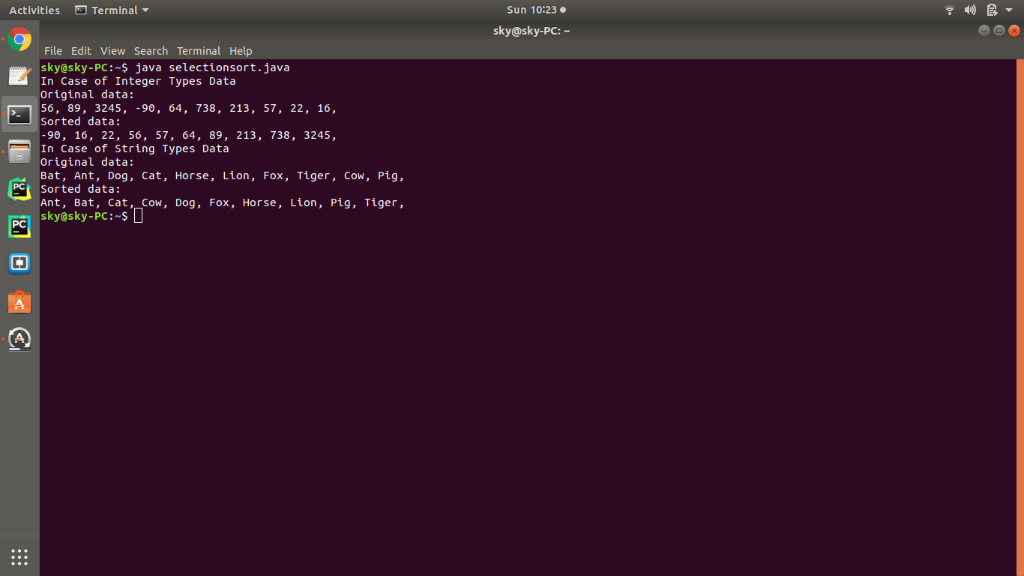# Java - Data Structures Q. Implement one of the sorts ( Selection Sort / Insertion Sort / Shell Sort / Merge Sort ) described in Chapters 8 and 9 . Input is an array with at least 10 items. The items c...

Java - Data Structures

Q.

Implement one of the sorts ( Selection Sort / Insertion Sort / Shell Sort / Merge Sort ) described in Chapters 8 and 9 . Input is an array with at least 10 items.

The items can be of any type (Suggested types are integers—denoting how many patrons visited the library in the last three weeks or strings—denoting the names of books returned today). Print the original data. Print the sorted data.

SOURCE CODE

public class Main
{
public static void selectionSort (String[]array)
{
System.out.println ("Original data: ");
for (String str:array)
System.out.print (str + ", ");
System.out.println ();

for (int j = 0; j < array.length - 1; j++)
{
int min = j;
for (int k = j + 1; k < array.length; k++)
if (array[k].compareTo (array[min]) < 0)
min = k;

String temp = array[j];
array[j] = array[min];
array[min] = temp;
}
System.out.println ("Sorted data: ");
for (String str:array)
System.out.print (str + ", ");
System.out.println ();
}
public static void selectionSort( int[] array )
{
System.out.println ("Original data: ");
for (int str:array)
System.out.print (str + ", ");
System.out.println ();

for ( int j=0; j < array.length-1; j++ )
{
int min = j;
for ( int k=j+1; k < array.length; k++ )
if ( array[k] < array[min] ) min = k;

int temp = array[j];
array[j] = array[min];
array[min] = temp;

}

System.out.println ("Sorted data: ");
for (int str:array)
System.out.print (str + ", ");
System.out.println ();

}

public static void main (String[]args)
{
int [] intArray = { 56,89,3245,-90,64,738,213,57,22,16 };
String[] strArray = { "Bat", "Ant", "Dog", "Cat", "Horse", "Lion", "Fox", "Tiger", "Cow", "Pig" };
System.out.println ("In Case of Integer Types Data");
selectionSort (intArray);
System.out.println ("In Case of String Types Data");
selectionSort (strArray);

}
}

OUTPUT

In Case of Integer Types Data
Original data:
56, 89, 3245, -90, 64, 738, 213, 57, 22, 16,
Sorted data:
-90, 16, 22, 56, 57, 64, 89, 213, 738, 3245,
In Case of String Types Data
Original data:
Bat, Ant, Dog, Cat, Horse, Lion, Fox, Tiger, Cow, Pig,
Sorted data:
Ant, Bat, Cat, Cow, Dog, Fox, Horse, Lion, Pig, Tiger,

Screenshot